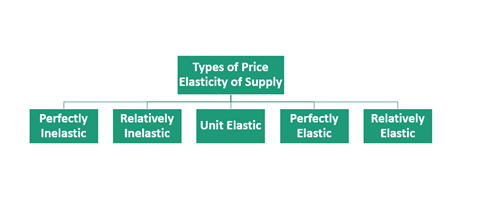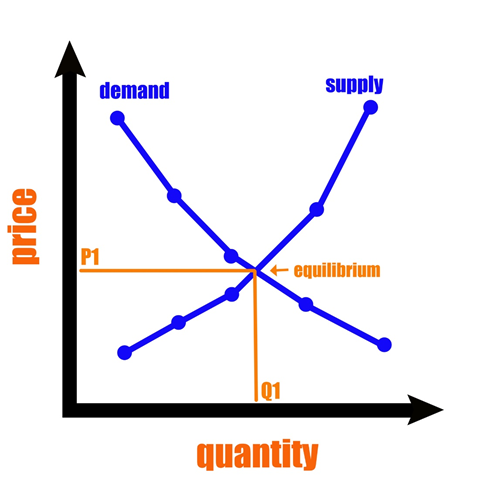# Price Elasticity of Supply

How do you define Price Elasticity of Supply?

Price elasticity of supply is a method used in economics to measure how the supply of goods and services changes with a change in their market price. It is a method to calculate the response of one variable (the supply of goods and services) to a change in another variable (price). As per the basic economic theory, an increase in the price of goods and services will also increase their supply. Contrarily, there will be a decrease in the supply of goods when their price decreases. Numerically, the price elasticity of supply (PES) is depicted as the percentage change in the supply of goods and services divided by the percentage change in price.

Price elasticity of supply can be denoted as PES or Es.

The formula to calculate price elasticity of supply is:

Es = Δy/Δx, where

Δy = % Change in Supply,

Δx = % Change in Price

Summary
• Price elasticity of supply is a method used in economics to measure how the supply of goods and services changes with a change in their market price.
• Numerically, the price elasticity of supply (PES) is depicted as percentage change in quantity supplied divided by the percentage change in price.
• As per the basic economic theory, an increase in the price of goods and services will also increase their supply. Contrarily, there will be a decrease in the supply of goods when their price decreases.

How does Price Elasticity Affect Supply ?

## What is elasticity?

In economics, elasticity refers to the percentage change in one economic variable in response to a change in another variable. In business, elasticity indicates the degree to which the consumers change their demand for the goods and services in response to a change in their price.

Overall, price elasticity calculates the change in demand or supply of goods and services in response to a change in prices. Thus, elastic indicates that a product is sensitive to any change in the price, whereas inelastic means that a product remains unaffected by the price movements.

How does an increase in demand for goods and services affect their price in the market?Image Source: © Rummess | Megapixl.com

As we know, producers compete for gains in a free market economy. Because the profits are never the same at all times for different kinds of goods, entrepreneurs tend to shift resources and the workforce efforts towards those products that can help them earn more profits. Thus, they move away from goods and focus less on less profitable goods. This results in an increased supply of highly valued goods and a reduction or decrease in the supply for goods that are considered less valuable. Economists and financial analysts term the tendency price and quantity supplied as related to the law of supply. For example, let us assume that suddenly consumers start demanding more apples and fewer mangoes. Thus, that there is more dollar bidding for apples and lesser for mangoes. This will result in an increase in apple prices and a decline in the price of mangoes. Observing this shift in the consumers' demand, the producers of fruits will decide to grow more apples and fewer mangoes since it can increase profits.

## What are the different types of Price Elasticity of Supply (PES)?

Furthermore, there are five kinds of price elasticity of supply (PES). They are perfectly inelastic, relatively inelastic, unit elastic, perfectly elastic, and relatively elastic.The PES has a range of values, which tells us whether a supply is elastic or inelastic. The different values of price elasticity of supply are discussed below:

• PES > 1: When the supply is relatively elastic.
• PES = 1: When the supply is unit elastic. When the PES value is equal to 1, the quantity supplied changes by an equal percentage as the price change.
• PES = 0: When the price elasticity of supply is equal to zero, the supply curve is vertical. This suggests that the quantity supplied remains unaffected by the change in prices. It means that the supply here is “perfectly inelastic.”
• PES = ∞ (infinity): When the value is infinity, it means that the supply curve is horizontal. It suggests that there is an extreme change in demand in response to even a minute change in prices. Thus, supply here is “perfectly elastic.”
• PES is between 0 and 1 = When the supply is relatively inelastic. This suggests that the percentage change in the number of goods and services supplied changes by a lower percentage than the price change percentage.

## Give examples of products exhibiting elastic and inelastic supply characteristics?

Let us take the example of the burger. Let us assume that an increase of 50% in burger price leads to an increase in the burger supply by 35%. Then, we can tell whether the supply is inelastic or elastic with the formula for price elasticity.

As we know, PES = % change in quantity supplied / % change in price.

PES = 35 % / 50 %

= 0.7. Since, the value is less than 1, it suggests that the burger exhibits inelastic supply characteristics.

Example which exhibits elastic supply characteristics

Say, for instance, a vending machine has been installed by a company for supplying Coco-Cola. Currently, the vending machines sell a bottle of Coco-Cola at \$3.50. At this price, a manufacturer supplies 4,000 bottles every week. However, due to some restrictions imposed by the government, the price has come down to \$3.00, which has reduced the supply of the number of Coco-Cola bottles to 3,000 per week.

Here the price elasticity of supply can be obtained by using the formula:

Now, change in supply = 3,000 - 4,000 (Coco Cola bottles) Change in Price = \$3.00 - \$3.50

## Therefore,

As we know, Price elasticity of supply formula = (3,000 – 4,000) / (3,000 + 4,000) ÷ (\$3.00 – \$3.50) / (\$3.00 + \$3.50)

= (-1/7) ÷ (-1/13)

= 13/7, which is 1.857

Therefore, here the Coco-Cola supplier exhibited elastic supply characteristics.

What are the determinants of Price Elasticity of Supply?Image Source: © Icefields | Megapixl.com

In the example discussed above, the answer to the degree of increase or decrease in the supply of apples and mangoes depends on these two fruits' elasticity of supply. This means that the supply of apples will increase drastically if they have an extremely high price elasticity of supply. Meanwhile, on the other hand, the supply of mangoes will not decline dramatically because they might possess a lower price elasticity of demand.

Numerous factors can affect price elasticity. Some of them are

1. The capacity to which industry can increase or decrease the production of a product that it already has.
2. Quantity of current stock, inventory, or raw materials that an industry owns.
3. The time taken by an industry to produce a product and the workforce and funds available also impact the quantity supplied.

## How can companies boost the Price Elasticity of Supply (PES)?

Usually, in remain agile with the shift in the price of products, companies try to keep the price elasticity of the supply high.

They try to profit when the price increases, whereas trim down the production when the price declines. However, companies can do a lot of things to boost the price elasticity of supply, such as:

• Technological upgradation like upgrading software and other equipment to enhance efficiency.
• Improved capacity and capable workforce.
• Expansion of storage facilities ensuring that the stored products last long.
• Improvement in the distribution and shipping of the products.

##### Top ASX Listed Companies

Definition:
We use cookies to ensure that we give you the best experience on our website. If you continue to use this site we will assume that you are happy with it. OK